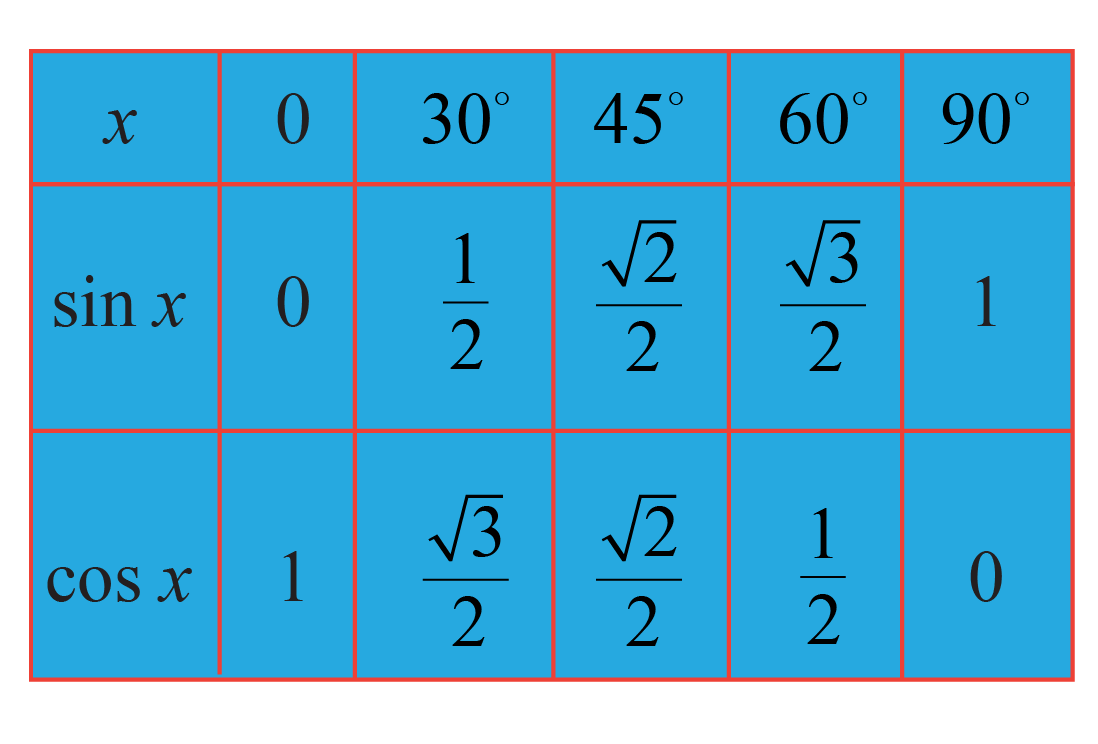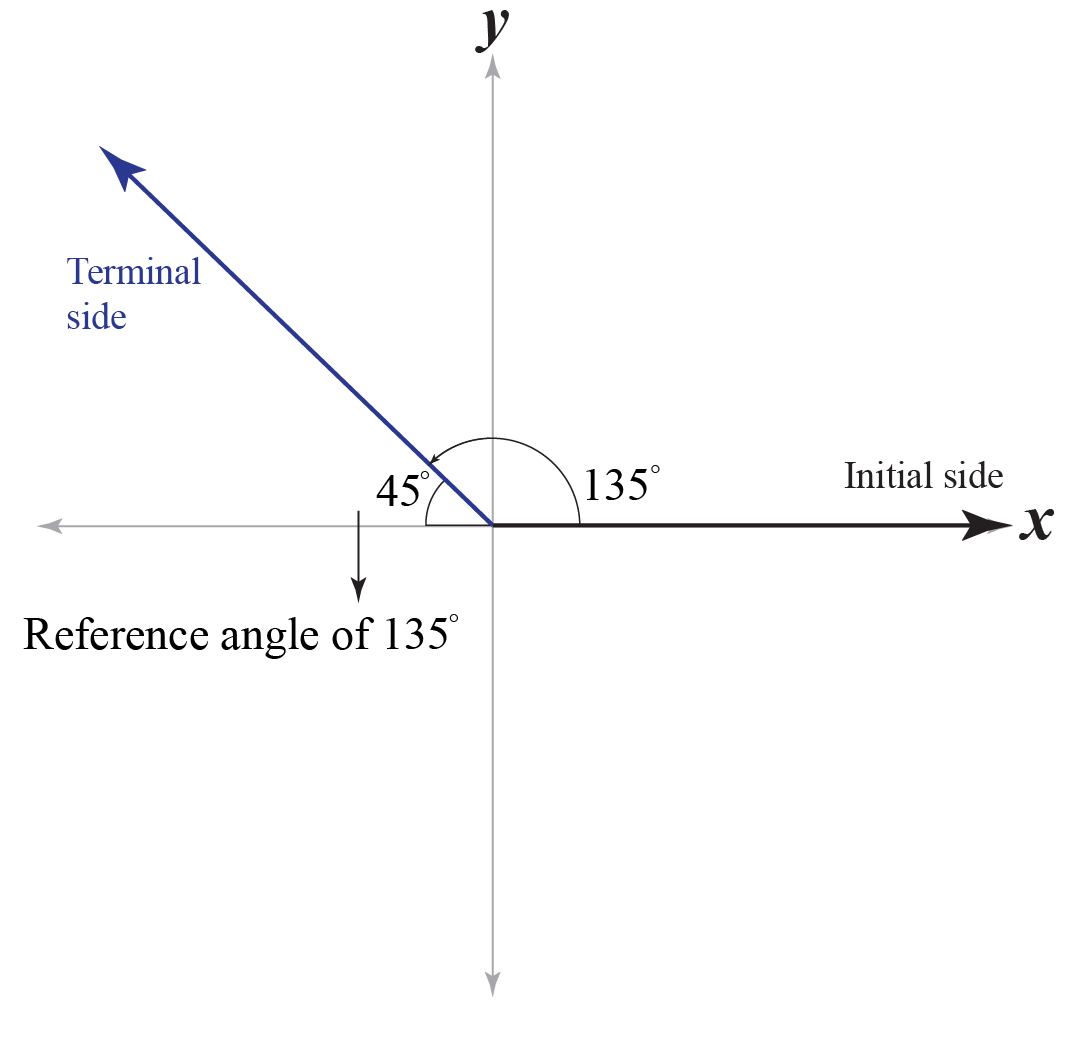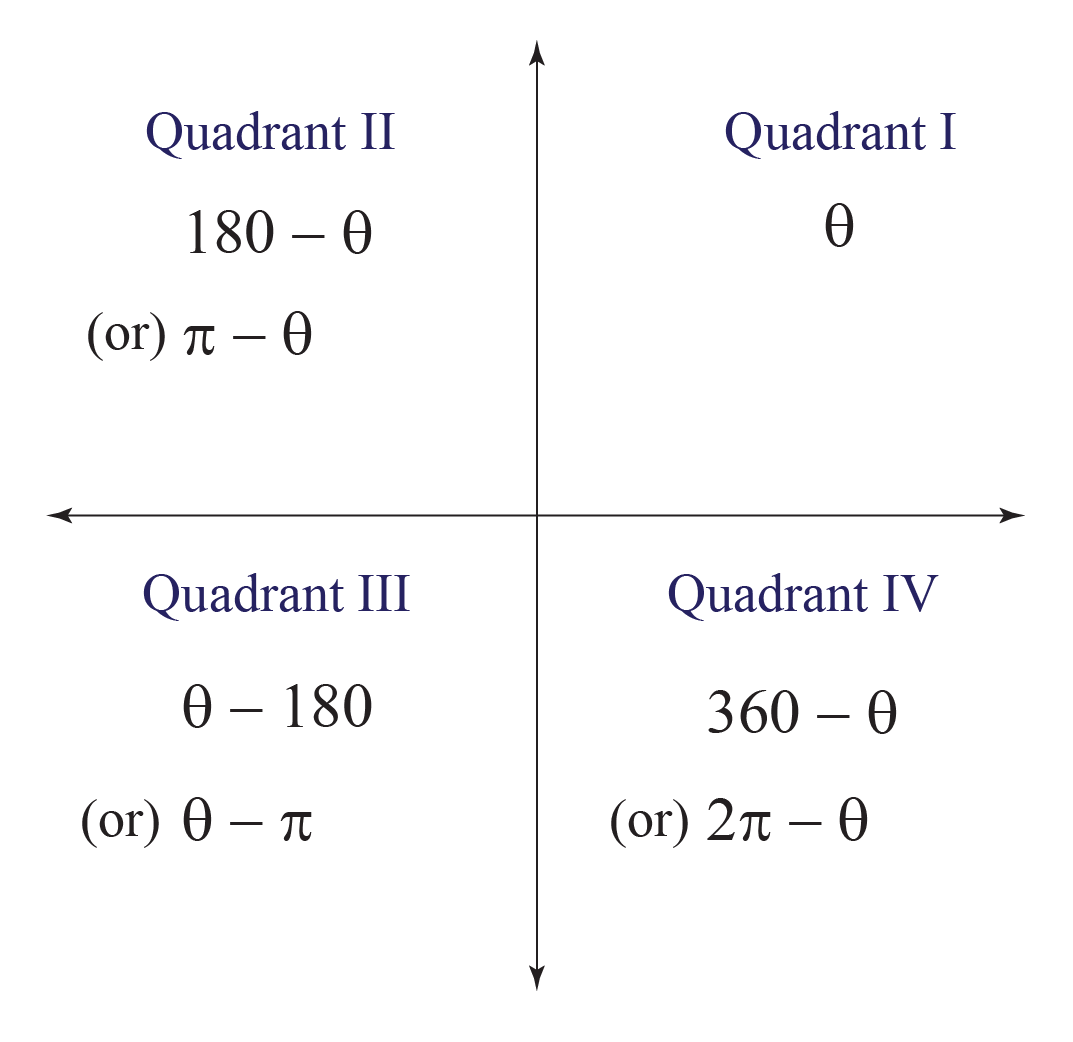# Reference Angle

Reference Angle

Ava was asked to find the value of $$\sin 45^\circ$$ and $$\sin 135^\circ$$. She uses the trigonometric table shown below to determine its value as $$\dfrac{\sqrt 2}{2}$$.She finds it hard to solve this problem because the value of 135o is not present in the table. Her friend explains to her that this can be solved using the reference angle of 135o

But do you know what is a reference angle? Let's learn all about it in this short lesson.

## Lesson Plan

 1 What Is a Reference Angle? 2 Important Notes on Reference Angle 3 Tips and Tricks on Reference Angle 4 Solved Examples 5 Interactive Questions

## What Is a Reference Angle?

The reference angle is the smallest possible angle made by the terminal side of the given angle with the x-axis.

### How to Draw Reference Angle?

To draw the reference angle for an angle, identify its terminal side and see by what angle the terminal side is close to the x-axis.

The reference angle of 135o is drawn below:Here, 45o is the reference angle of 135o

## What Are the Rules for Reference Angles in Each Quadrant?

Here are the reference angle formulas depending on the quadrant of the given angle.

Angle, $$\theta$$ Reference angle
I lies between 0o and 90o

$$\theta$$

II lies between 90o and 180o

$$180-\theta$$

III lies between 180o and 270o

$$\theta-180$$

IV lies between 270o and 360o

$$360-\theta$$

If the angle is in radians, then we use the same rules above by replacing 180o with $$\pi$$ and 360o with $$2\pi$$.Example

Find the reference angle of 135o.

Solution

The given angle is, $$\theta=135^\circ$$

We know that 135o lies in quadrant II.

Using the above rules, its reference angle is,

$180-\theta=180-135 = 45^\circ$

Therefore, the reference angle of 135o is 45o.

## How to Find Reference Angles?

In the previous section, we learned that we could find the reference angles using the set of rules mentioned in the table.

That table works only when the given angle lies between 0o and 360o.

But what if the given angle does not lie in this range?

Let's see how we can find the reference angles in this case.

### Steps to Find the Reference Angles

The steps to find the reference angle of an angle is explained with an example.

Example

Find the reference angle of 480o.

Solution

Step 1: Find the coterminal angle of the given angle that lies between 0o and 360o.

The coterminal angle can be found either by adding or subtracting 360o from the given angle as many times as required.

Let's find the coterminal angle of 480o that lies between 0o and 360o.

We will subtract 360o from 480o to find its coterminal angle.

$480^\circ - 360^\circ = 120^\circ$

Step 2: If the angle from step 1 lies between 0o and 90o, then that angle itself is the reference angle of the given angle.

Else, we have to check whether it is closest to 180o or 360o and by how much.

Here, 120o does not lie between 0o and 90o

Also, 120o is closest to 180o by 60o. i.e.,

$180^\circ - 120^\circ = 60^\circ$

Step 3: The angle from step 2 is the reference angle of the given angle.

Thus, the reference angle of 480o is 60o.Tips and Tricks
1. We use the reference angle to find the values of trigonometric functions at an angle that is beyond 90o.
For example, we can see that the coterminal angle and reference angle of 495o are 135o and 45o respectively.

\begin{align}&\sin 495^\circ \0.2cm] &= \sin 135^\circ \\[0.2cm] &= +\sin 45^\circ \end{align} We have included the + sign because 135o is in quadrant II, where sine is positive. \begin{align}\sin 495^\circ = \dfrac{\sqrt 2}{2}\,\,\, \text{ [Using unit circle]} \end{align} 2. If we use reference angles, we don't need to remember the complete unit circle, instead we can just remember the first quadrant values of the unit circle. ## Reference Angle Calculator Here is the reference angle calculator. You can enter any angle here and it will give the corresponding reference angle with step-by-step explanations. ## Solved Examples  Example 1 Find the reference angle of $$\dfrac{8\pi}{3}$$ Solution The given angle is greater than $$2\pi$$ Finding coterminal angle We find its coterminal angle by subtracting $$2\pi$$ from it. \[\dfrac{8\pi}{3} - 2\pi = \dfrac{2\pi}{3}

This angle does not lie between 0 and $$\dfrac{\pi}{2}$$. Hence, it is not the reference angle of the given angle.

Finding reference angle

Let's check whether $$\dfrac{2\pi}{3}$$ is close to $$\pi$$ or $$2\pi$$ and by how much.

Clearly, $$\dfrac{2\pi}{3}$$ is close to $$\pi$$ by $\pi-\dfrac{2\pi}{3} = \dfrac{\pi}{3}$

Therefore,

 $$\therefore$$ The reference angle of $$\dfrac{8\pi}{3}$$ is $$\dfrac{\pi}{3}$$
 Example 2

Using the above example (Example 1), find $$\cos \dfrac{8\pi}{3}$$.

Solution

In the above example, we found that the coterminal angle of $$\dfrac{8\pi}{3}$$ is $$\dfrac{2\pi}{3}$$ which lies in quadrant II, where cos is negative.

$\cos \dfrac{8\pi}{3} = - \cos \dfrac{2\pi}{3}$

Also, we found that the reference angle of $$\dfrac{8\pi}{3}$$ is $$\dfrac{\pi}{3}$$. So

\begin{align}\cos \dfrac{8\pi}{3} &= - \cos \dfrac{2\pi}{3}\\[0.2cm] &= - \cos \dfrac{\pi}{3}\\[0.2cm] &= - \dfrac{1}{2}\,\,\,\,\,\text{ (Using the trigonometry table)} \end{align}

Therefore,

 $$\therefore$$$$\cos \dfrac{8\pi}{3} = - \dfrac{1}{2}$$
 Example 3

Find the reference angle of -1200o.

Solution

The given angle is  -1200o.

Finding the coterminal angle

We see that $\dfrac{1200}{360}= 3.333...$

This indicates that there are 3 complete angles (360o angles) in 1200o.

To find a positive coterminal angle of -1200o, we need to add 4 multiples of 360o to it.

(If we add just 3 multiples of 360o to it, the answer won't be a positive angle).

$-1200^\circ + 4(360^\circ) = 240^\circ$

Finding the reference angle

Let's check whether 240o is close to 180o or 360o and by how much.

Clearly, 240is close to 180o and by 60o

Therefore,

 $$\therefore$$ The reference angle of -1200o is 60o

## Interactive Questions

Here are a few activities for you to practice.

## Let's Summarize

We hope you enjoyed learning about the reference angle with the simulations and practice questions. Now, you will be able to easily solve problems on reference angle formula, reference angle calculator, negative reference angle, and how to draw reference angle.

At Cuemath, our team of math experts is dedicated to make learning fun for our favorite readers, the students! Through an interactive and engaging learning-teaching-learning approach, the teachers explore all angles of a topic. Be it problems, online classes, videos, or any other form of relation, it’s the logical thinking and smart learning approach that we at Cuemath believe in.

## 1. What is the reference angle for a 200o angle?

Between the angles 180o and 360o, we can say that 200o is close to 180o by 20o

Thus, the reference angle of 200o is 20o

## 2. What is the reference angle for a 275o angle?

Between the angles 180o and 360o, we can say that 275o is close to 360o by 85o

Thus, the reference angle of 275o is 85o

## 3. What is the reference angle for a 235o angle?

Between the angles 180o and 360o, we can say that 235o is close to 180o by 55o.

Thus, the reference angle of 235o is 55o

More Important Topics
Numbers
Algebra
Geometry
Measurement
Money
Data
Trigonometry
Calculus
More Important Topics
Numbers
Algebra
Geometry
Measurement
Money
Data
Trigonometry
Calculus